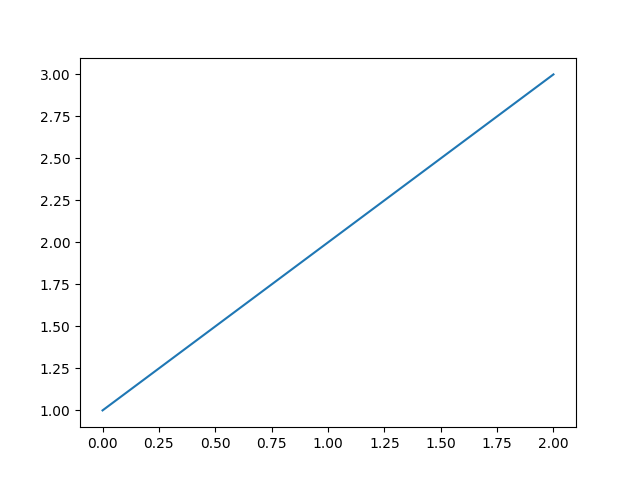Travis-CI:# Agg Buffer¶

Use backend agg to access the figure canvas as an RGB string and then convert it to an array and pass it to Pillow for rendering.```import numpy as np

import matplotlib.pyplot as plt
from matplotlib.backends.backend_agg import FigureCanvasAgg

try:
from PIL import Image
except ImportError:
raise SystemExit("Pillow must be installed to run this example")

plt.plot([1, 2, 3])

canvas = plt.get_current_fig_manager().canvas

agg = canvas.switch_backends(FigureCanvasAgg)
agg.draw()
s = agg.tostring_rgb()

# get the width and the height to resize the matrix
l, b, w, h = agg.figure.bbox.bounds
w, h = int(w), int(h)

X = np.fromstring(s, np.uint8).reshape((h, w, 3))

try:
im = Image.fromstring("RGB", (w, h), s)
except Exception:
im = Image.frombytes("RGB", (w, h), s)

# Uncomment this line to display the image using ImageMagick's
# `display` tool.
# im.show()
```

Total running time of the script: ( 0 minutes 0.077 seconds)

Gallery generated by Sphinx-Gallery